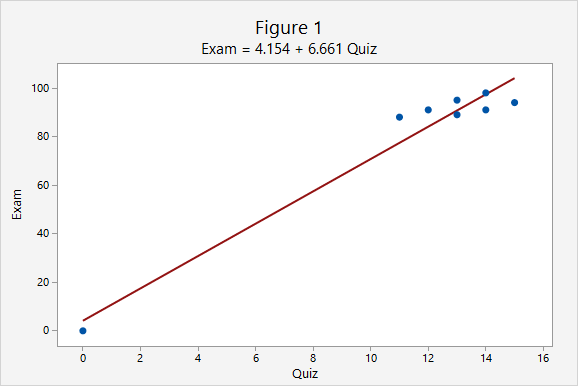# 3.4.2 - Correlation

In this course, we will be using Pearson's $$r$$ as a measure of the linear relationship between two quantitative variables. In a sample, we use the symbol $$r$$. In a population, we use the Greek letter $$\rho$$ ("rho"). Pearson's $$r$$ can easily be computed using statistical software.

Correlation
A measure of the direction and strength of the relationship between two variables.
Properties of Pearson's r
1. $$-1\leq r \leq +1$$
2. For a positive association, $$r>0$$, for a negative association $$r<0$$, if there is no relationship $$r=0$$
3. The closer $$r$$ is to $$0$$ the weaker the relationship and the closer to $$+1$$ or $$-1$$ the stronger the relationship (e.g., $$r=-0.88$$ is a stronger relationship than $$r=+0.60$$); the sign of the correlation provides direction only
4. Correlation is unit free; the $$x$$ and $$y$$ variables do NOT need to be on the same scale (e.g., it is possible to compute the correlation between height in centimeters and weight in pounds)
5. It does not matter which variable you label as $$x$$ and which you label as $$y$$. The correlation between $$x$$ and $$y$$ is equal to the correlation between $$y$$ and $$x$$.

The following table may serve as a guideline when evaluating correlation coefficients:

Absolute Value of $$r$$ Strength of the Relationship
0 - 0.2 Very weak
0.2 - 0.4 Weak
0.4 - 0.6 Moderate
0.6 - 0.8 Strong
0.8 - 1.0 Very strong
Cautions
1. Correlation does NOT equal causation. A strong relationship between $$x$$ and $$y$$ does not necessarily mean that $$x$$ causes $$y$$. It is possible that $$y$$ causes $$x$$, or that a confounding variable causes both $$x$$ and $$y$$.
2. Pearson's $$r$$ should only be used when there is a linear relationship between $$x$$ and $$y$$. A scatterplot should be constructed before computing Pearson's $$r$$ to confirm that the relationship is not non-linear.
3. Pearson's $$r$$ is not resistant to outliers. Figure 1 below provides an example of an influential outlier. Influential outliers are points in a data set that increase the correlation coefficient. In Figure 1 the correlation between $$x$$ and $$y$$ is strong ($$r=0.979$$). In Figure 2 below, the outlier is removed. Now, the correlation between $$x$$ and $$y$$ is lower ($$r=0.576$$) and the slope is less steep.Note that the scale on both the x and y axes has changed. In addition to the correlation changing, the y-intercept changed from 4.154 to 70.84 and the slope changed from 6.661 to 1.632.